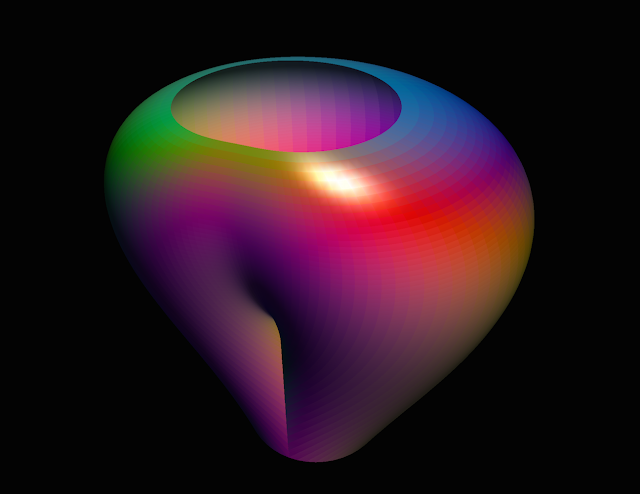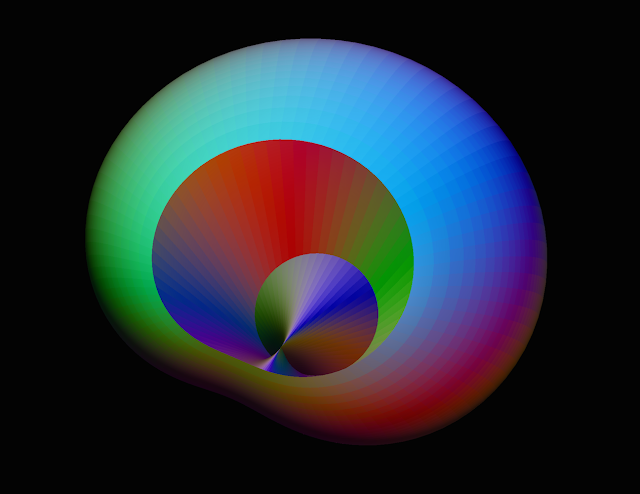## Wednesday, September 9, 2015

### Some Cool Views of Projective Space

While finally revisiting one of the geometry books on my shelf, Glen Bredon's Topology and Geometry, I encountered an exercise about showing that the projective space is homeomorphic to the mapping cone of a map that doubles a circle on itself (the complex squaring map $z \mapsto z^2$). The mapping cone has a nice visualization, first as a mapping cylinder, which takes a space $X$ and crosses it with the interval $I$ to form $X \times I$ (thus forming a "cylinder"), and then glues the bottom of it to another space $Y$ using a given continuous map $f : X \to Y$. Finally, to make the cone, it collapses the top to a single point. Of course, this can be visualized as deforming the bottom part of $X \times I$ through whatever contortion $f$ does, which might include self-intersection (and of course, it could be more gradual). So I used a good old friend, parametrizations, to help set up an explicit example. Take a look!An immersion of projective space into $\mathbb R^3$. Shown as a mesh to make the self-intersecting portion visible. Looks a little like a molar, although one would hope I take good enough care of my teeth to not have that many holes in it…A cutaway view, now as a more solid surface, basically illustrating it now as a mapping cylinder (it is homeomorphic to projective space minus a disk, which is a Möbius strip). Anyone know a good glassblower so we can make vases that look like this?A view from the open top, allowing us to see the self-intersecting part of the surface from above
The equation, in "cylindrical coordinates", is $r = (2z+\cos\theta) \sqrt{1-z^2}$ for $0\leq z \leq 1$ (for the closed surface), $0 \leq z \leq 0.97$ (for the cutaway), and $0 \leq \theta \leq$ (what else?) $2\pi$. I say it in scare quotes because technically, it allows negative values of $r$. For fun, though (and to make it totally legit, even if you have qualms about negative radii), we rewrite it as a (Cartesian) parametrization (by substituting for $r$):
$\begin{pmatrix} x \\ y \\ z\end{pmatrix} = \begin{pmatrix} (2u + \cos v)\sqrt{1-u^2} \cos v\\ (2u + \cos v) \sqrt{1-u^2}\sin v \\ u\end{pmatrix}$
with $0\leq u \leq 1$ or $0.97$, and $0 \leq v \leq 2\pi$.

The motivation for this is that the equations $r = a + \cos\theta$, as $a$ varies, goes from a loop traversed once to a loop that folds over itself exactly in a $2:1$ manner:

but now visualized stacked on top of one another. Then to collapse the top, we shrink the diameter by a function that vanishes at $1$ and approaches $0$ at infinite slope to make it smooth (topologically speaking, it is valid to let it collapse to a corner point, though). Flowers, however, are not included (you'll have to fiddle with things like $r = \cos(k\theta)$ if you want that…)